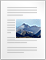# Vector Algebra 2

In this post we’ll lern Vector algebra in component form.
Component of any vector is the projection of that vector along the three coordinate axis X, Y, Z.

In component form addition of two vectors is
C = (Ax+ Bx)i + (Ay+ By)j + (Ay+ By)k
where,
A = (Ax, Ay, Az) and B = (Bx, By, Bz)
Thus in component form resultant vector C becomes,
Cx = Ax+ Bx
Cy = Ay+ By
Cz = Az+ Bz

SUBTRACTION OF TWO VECTORS
In component form subtraction of two vectors is
D = (Ax– Bx)i + (Ay– By)j + (Ay– By)k
where,
A = (Ax, Ay, Az) and B = (Bx, By, Bz)
Thus in component form resultant vector D becomes,
Dx = Ax – Bx
Dy = Ay– By
Dz = Az– Bz

NOTE:- Two vectors add or subtract like components.

DOT PRODUCT OF TWO VECTORS
A.B = (Axi + Ayj + Azk) . (Bxi + Byj + Bzk)
= AxBx + AyBy + AzBz.
Thus for calculating the dot product of two vectors, first multiply like components, and then add.

CROSS PRODUCT OF TWO VECTORS

A x B = (Axi + Ayj + Azk) x (Bxi + Byj + Bzk)
= (AyBz – AzBy)i + (AzBx – AxBz)j + ( AxBy – AyBx)k.

Cross product of two vectors is itself a vector.
To calculate the cross product, form the determinantwhose first row is x, y, z, whose second row is A (in component form), and whose third row is B.

VECTOR TRIPPLE PRODUCT

Vector product of two vectors can be made to undergo dot or cross product with any third vector.

(a) Scalar tripple product:-
For three vectors A, B, and C, their scalar triple product is defined as
A . (B x C) = B . (C x A) = C . (A x B)
obtained in cyclic permutation. If A = (Ax, Ay, Az) , B = (Bx, By, Bz) , and C = (Cx, Cy, Cz) then A . (B x C) is the volume of a parallelepiped having A, B, and C as edges and can easily obtained by finding the determinant of the 3 x 3 matrix formed by AB, and C.

(b) Vector Triple Product:-
For vectors A, B, and C, we define the vector tiple product as
A x (B x C) = B(A . C) – C(A – B)
Note that
(A . B)C is not equal to A(B . C)
but
(A . B)C = C(A . B).Vector algebra Synopsis (118.6 KiB)